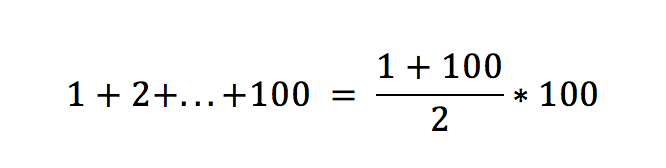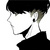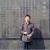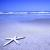【C语言】1到100求和，原来还可以这样写？

【推荐阅读】滴滴出行的数据架构和信息流处理思路解析->>>#include <stdio.h>

int main(){

int a1 = 1;

int a2 = 2;

int sum =a1 + a2;

return 0;

}

#include <stdio.h>

int main(){

int a1 = 1;

int a2 = 2;

...

int a100 =100;

int sum =a1 + a2+...+a100;

return 0;

}

（上面的代码并不是完整的代码，其中的省略号部分需要大家自己补全。）

int i = 0;

for( i = 1; i <= 100; i++)

int i = 0;

int sum = 0;

for(i = 1; i <= 100; i++){

sum = sum +i;

}int sum = (1+100)*100 / 2;

00